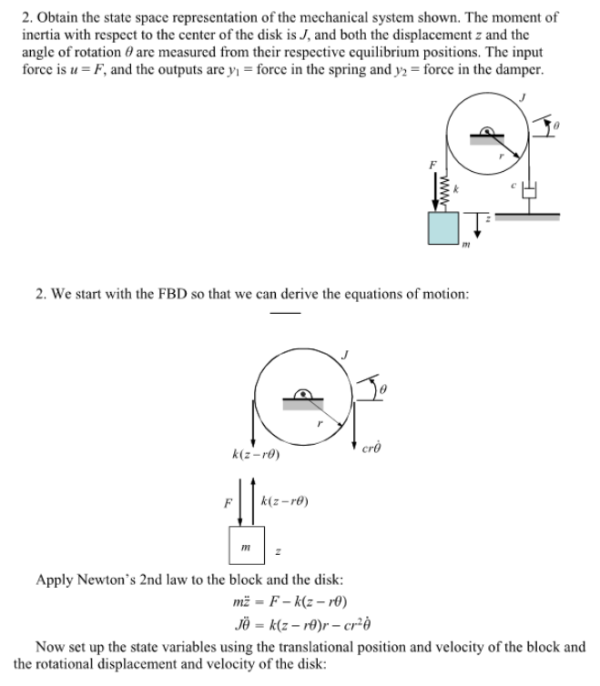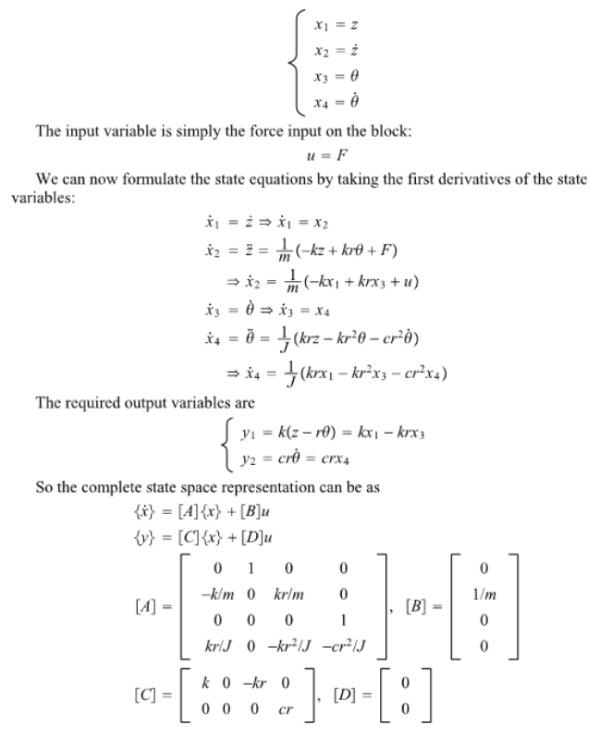Obtain the state space representation of the mechanical system shown. The moment of inertia with respect to the center of the disk is J, and both the displacement z and the angle of rotation theta are measured from their respective equilibrium positions. The input force is u=F, and the outputs are y1=force in the spring and y2=force in the damper.Obtain the state space representation of the mechanical system shown. The moment of inertia with respect to the center of the disk is J, and both the displacement z and the angle of rotation theta are measured from their respective equilibrium positions. The input force is u=F, and the outputs are y1=force in the spring and y2=force in the damper.

System Dynamics Page 2 dynamics dynamics dynamics dynamics dynamics dynamics dynamics System dynamics Page 3